UPSC  >  Sets based Questions

# Sets based Questions - CSAT Preparation - UPSC

A venn diagram is used to visually represent the relationship between various sets.

What do each of the areas in the figure represent?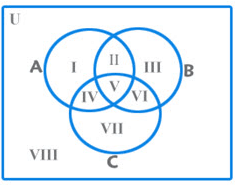I – only A;

II – A and B but not C;

III – Only B;

IV – A and C but not B;

V – A and B and C;

VI – B and C but not A;

VII – Only C

n(A U B U C) = n(A) + n(B) + n(C) — n(A n B) — n(A n C) – n(B n C) + n(A n B n C)

As per the above diagram,

n(A) = I + II + IV + V

n(B) = II + III + V + VI

n(C) = IV + V + VI + VII

n(A n B) = II + V

n(B n C) = V + VI

n(C n A) = IV + V

n(A n B n C) = V

n(A U B U C) = I + II + III + IV + V + VI + VII

Note: While doing such questions, it is advisable that you take the least no. of variables to fill up the empty space. As a practice, if n(A n B n C) is missing, take that as ‘x’ and proceed.

For Maximum and Minimum of values, the key point to note is:

If you allot a value to the intersection, it will get added to all the individual sets but will bring down the total.

Example: In a survey, it was found that 80% like tea whereas 70% like coffee. What is the maximum and the minimum number of those who like both?
Ans: First thing to note is that no information is mentioned about the people who don’t like either of them. So that value is flexible and can change.
n(tea) = 80
n(coffee) = 70

n(total) = 100 {This includes those who like neither.}

n(tea n coffee) = ??? {We don’t know this value and it is flexible}
If we want to maximize those who like both, we have to maximize the value in the intersection. So, we have to minimize the value of the union.

n(tea n coffee)max = 70 {It is limited by the higher of the two values}

In this case, our Venn diagram will look something like this (Red is tea & Purple is coffee):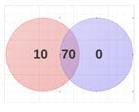In this case 20% of people like neither tea nor coffee.

If we want to minimize those who like both, we have to minimize the value in the intersection. So, we have to maximize the value of the union.  We know that the maximum possible value of the union ie n(tea U coffee) = 100

So, we need to figure out the surplus  : n(tea) + n(coffee) = 80 + 70 = 150.

The surplus is = 150 – 100 = 50

So, the value of the intersection = value of the surplus = 50

This could have also been obtained by the formula

n(a U b) = n(a) + n(b) – n (a n b)
In this case, our Venn diagram will look something like this (Red is tea & Purple is coffee):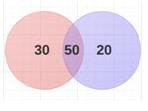In this case, there is no one who likes neither coffee nor tea.
Let us look at a slightly more complicated problem when we have to deal with three sets and the value of the union of the sets is fixed.

Example: In a survey, it was found that 40 % like tea, 50 % like coffee and 60 % like milk. Every person likes at least one of the three items – tea/coffee/milk. What are the maximum and minimum possible values of those who like all three?
Solution: Currently our Venn Diagram looks like this: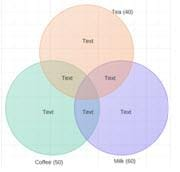First of all, we need to figure out the surplus.

Surplus = 40 +50 + 60 – 100 = 50

The surplus should be taken care of by adding to the intersection of all three or any of the two.

If we want to maximize those who like all three, we need to maximize the intersection of all three. Adding ‘1’ to the intersection of all three takes care of a surplus of ‘2’. To take care of a surplus of 50, we need to make
n (tea n coffee n milk) = 25
Note: If the union of the sets was not fixed i.e. the line ‘Every person likes at least one of the three items – tea/coffee/milk’ was not given in the question then the answer would have been 40.
Our Venn diagram will now look like this: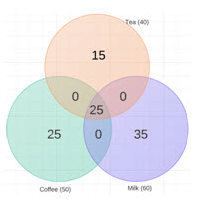If we want to minimize those who like all three, we need to minimize the intersection of all three. But we have to take care of the surplus of 50. We can do that by adding them to the intersection of any two of them. Adding ‘1’ to the intersection of two sets takes care of a surplus of ‘1’. So,

n (tea n coffee n milk)min = 0

We can take care of the surplus 50 in many ways by adding them in any order to the intersection of two sets. Three of those many ways are given below in Venn Diagrams.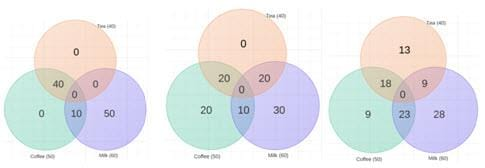The document Sets based Questions | CSAT Preparation - UPSC is a part of the UPSC Course CSAT Preparation.
All you need of UPSC at this link: UPSC

## CSAT Preparation

197 videos|151 docs|200 tests

## FAQs on Sets based Questions - CSAT Preparation - UPSC

 1. What are sets in mathematics?Ans. In mathematics, a set is a collection of distinct objects, considered as an object in its own right. These objects can be anything, such as numbers, letters, or even other sets.
 2. What is the cardinality of a set?Ans. The cardinality of a set is the number of elements it contains. It can be finite, meaning it has a specific number of elements, or infinite, meaning it has an uncountable number of elements.
 3. How are sets represented?Ans. Sets are often represented using curly braces {}. The elements of the set are listed inside the braces, separated by commas. For example, {1, 2, 3} represents a set with the elements 1, 2, and 3.
 4. What is the difference between a set and a subset?Ans. A set is a collection of elements, while a subset is a set that contains only elements from another set. In other words, all the elements of a subset are also elements of the original set.
 5. How are sets related to mathematical operations?Ans. Sets have various mathematical operations associated with them, such as union, intersection, and complement. These operations allow us to combine sets, find common elements, or identify elements that are not in a particular set. These operations are useful for solving problems in different branches of mathematics.

## CSAT Preparation

197 videos|151 docs|200 testsExplore Courses for UPSC exam### How to Prepare for UPSC

Read our guide to prepare for UPSC which is created by Toppers & the best Teachers
Signup to see your scores go up within 7 days! Learn & Practice with 1000+ FREE Notes, Videos & Tests.
10M+ students study on EduRev
Track your progress, build streaks, highlight & save important lessons and more!
Related Searches

,

,

,

,

,

,

,

,

,

,

,

,

,

,

,

,

,

,

,

,

,

;